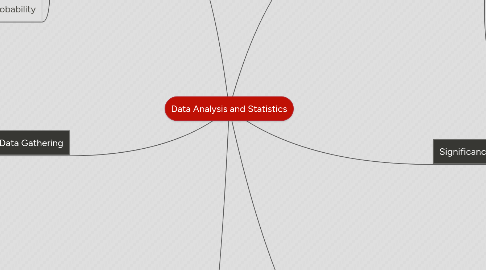# Data Analysis and StatisticsJoshua Hoffer
Get Started. It's FreeData Analysis and Statistics## 1. Binomial Distributions

### 1.2. Binomial Theorem

1.2.1. The formula for finding any power of a binomial without multiplying at length

### 1.3. Binomial Experiment

1.3.1. Consists of n independent trial whose outcomes are either successes or failures

1.3.2. The probability of success p is the same for each trial, and the probability of failure q is the same for each trial

1.3.3. Ex: 10 flips of a coin                            Success: Heads                                  Failure: Tails                                       P(success):p=0.5                     P(failure): q=1-p=0.5

### 1.4. Binomial Probability

1.4.1. Probability that a binomial experiment results in exactly x successes

## 2. Sampling Distributions

### 2.1. Simple Random Sample

2.1.1. Members are chosen using a method that gives everyone an equally likely chance of being selected

### 2.2. Systematic Sample

2.2.1. Members are chosen using a pattern

### 2.3. Stratified Sample

2.3.1. The population is first divided into groups then members are randomly chosen from each group

### 2.4. Cluster Sample

2.4.1. The population is first divided into groups, a sample of the groups is randomly chosen, all members of the chosen

### 2.5. Convenience Sample

2.5.1. Members are chosen because they are easily accessible

### 2.6. Self-Selected

2.6.1. Members volunteer to participate

### 2.7. Probability Sample

2.7.1. Where every member of the population being sampled has a nonzero probability of being selected

### 2.8. Margin of Error

2.8.1. Defines an interval, centered on the sample percent, in which the population percent is most likely to lie

2.8.2. Ex: If the margin of error says plus or minus 3% 58% that means it will lie within 3% so the answer would be 55% or 61%

## 3. Data Gathering

### 3.1. Population

3.1.1. Entire group of people or objects that you want information about

### 3.2. Census

3.2.1. A survey of the entire population

### 3.3. Parameter

3.3.1. A number that describes the population

### 3.4. Statistic

3.4.1. A number that describes the sample

### 3.5. Sample

3.5.1. Random Sample

3.5.1.1. When every member has an equal chance of being selected

3.5.2. Biased Sample

3.5.2.1. A sample that does not represent the population

## 4. Surveys, Experiments, and Observational Studies

### 4.1. Experiment

4.1.1. Imposes on individuals to collect data on their response to the treatment

### 4.2. Observational Study

4.2.1. Observes individuals and measures variables without controlling the individuals or their environment in any way

### 4.3. Controlled Experiment

4.3.1. Two groups are studied under conditions that are identical except for one variable

### 4.5. Control Group

4.5.1. Does not receive the treatment

### 4.6. Randomized Comparative Experiment

4.6.1. Individuals are assigned to the control group or the treatment group at random, in order to minimize bias

## 5. Measures of Central Tendencies

### 5.1. Median

5.1.1. Middle value of the numbers in a date set

### 5.2. Mean

5.2.1. The average of numbers in a data set

### 5.3. Mode

5.3.1. The value that appears most often in a data set

### 5.4. Expected Value

5.4.1. The weighted average of all the possible outcomes

### 5.5. Box and Whisker Plot

5.5.1. Minimum

5.5.1.1. The smallest value in the data set

5.5.2. First Quartile

5.5.2.1. Median of the lower half of the data set

5.5.3. Third Quartile

5.5.3.1. Median of the upper half of the data set

5.5.4. Maximum

5.5.4.1. The largest value in the data set

5.5.5. Interquartile Range

5.5.5.1. The difference between the first and third quartile

### 5.6. Variance

5.6.1. The average of the squared differences from the mean

### 5.7. Standard Deviation

5.7.1. The square root of the variance

### 5.8. Outlier

5.8.1. A value that is much less or greater than the other data values

## 6. Significance of Experimental Results

### 6.1. Hypothesis Testing

6.1.1. Used to determine whether the difference in two groups is likely to be caused by chance

### 6.2. Null Hypothesis

6.2.1. States that there is no difference between the two groups being tested